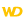Home |Sign in | FrançaisHelpWLanguageWLanguage syntaxData typeAdvanced typesThe different types of integersTypes of integersNotesSee alsoCharacter stringsConverting C typesCurrenciesDateDateTimeDurationJSONNumericOperations available for the dates, times and durations, ...RealsRectangleThe "Buffer" typeThe Variant typeThe different types of dataThe different types of integersTimeUUID
• Types of integers
• Different types of integers are available in WLanguage
• Notes
• Default value
• Thousand separator
• Differences between WINDEV 5.5 and WINDEV 25
WINDEVWEBDEVWINDEV MobileOthersThe different types of integers
Types of integers

Different types of integers are available in WLanguage

 Value included between Integer -2 x 109 and 2 x 109 (roughly)Example: i is inti = 5 1-byte integer -128 and 127 (inclusive)Example: i is 1-byte intThis type of variable will be automatically converted into Integer.This type of variable will be automatically converted into Integer.Versions 23 and laterThe 1-byte Integer variables are now available.New in version 23The 1-byte Integer variables are now available.The 1-byte Integer variables are now available. 2-byte integer -32.768 and 32.767 (inclusive)Example: I is 2-byte intThis type of variable will be automatically converted into Integer.This type of variable will be automatically converted into Integer.Versions 23 and laterThe 2-byte Integer variables are now available.New in version 23The 2-byte Integer variables are now available.The 2-byte Integer variables are now available. 4-byte integer -2 x 109 and 2 x 109 (roughly)Example: i is 4-byte int 8-byte integer -9 x 1018 and 9 x 1018 (roughly)Example: i is 8-byte int Unsigned integer 0 and 4 x 109Example: i is unsigned intThis type of variable will be automatically converted into Integer.Versions 16 and laterByteNew in version 16ByteByte 0 and 255 (inclusive)This type is equivalent to the unsigned 1-byte integer.Example: i is byteThis type of variable will be automatically converted into Integer. Unsigned 1-byte integer 0 and 255 (inclusive)Example: i is unsigned 1-byte intThis type of variable will be automatically converted into Integer. Unsigned 2-byte integer 0 and 65.535 (inclusive)Example: i is unsigned 2-byte intThis type of variable will be automatically converted into Integer. Unsigned 4-byte integer 0 and 4 x 109 (about)Example: i is unsigned 4-byte intThis type of variable will be automatically converted into Integer. Unsigned 8-byte integer 0 and 18 x 1018Example: i is unsigned 8-byte intThis type of variable will be automatically converted into Integer. System integer automatically adapts to the size supported by the compilation mode (4 bytes for a program compiled in 32 bits, 8 bytes for a program compiled in 64 bits). Example: i is system intVersions 22 and laterRemark: SystemIntegerToInteger is used to convert a system integer into a 4-byte integer.New in version 22Remark: SystemIntegerToInteger is used to convert a system integer into a 4-byte integer.Remark: SystemIntegerToInteger is used to convert a system integer into a 4-byte integer.
The exact values for these different types of integers can be found in the Limits.wl file found in the Personal\External sub-directory of the setup directory of WINDEV, WEBDEV and WINDEV Mobile.
Remark: All the advanced types (different from the "Integer" type) are recommended when using the Windows APIs.
Notes

Default value

An "Integer" variable that is declared but not initialized is equal to 0.

To enter an hexadecimal value in the code, the hexadecimal value must be prefixed by "0x".
MyVariable is int
MyVariable = 0x4D5Versions 23 and later
Remark: The '_' character can be used as block separator in the hexadecimal numbers. The blocks can have a size equal to 2, 4 or 8 characters long.
Example:
Nb1 is int = 0x_12_15_A4_BC
Nb2 is int = 0x_CD4A_DE58New in version 23
Remark: The '_' character can be used as block separator in the hexadecimal numbers. The blocks can have a size equal to 2, 4 or 8 characters long.
Example:
Nb1 is int = 0x_12_15_A4_BC
Nb2 is int = 0x_CD4A_DE58
Remark: The '_' character can be used as block separator in the hexadecimal numbers. The blocks can have a size equal to 2, 4 or 8 characters long.
Example:
Nb1 is int = 0x_12_15_A4_BC
Nb2 is int = 0x_CD4A_DE58Versions 23 and later

Thousand separator

The 'Space' character or the '_' character can be used as thousand separator in the numbers. For example:
num1 is int
num1 = 123 456
let num2 = 246_789New in version 23

Thousand separator

The 'Space' character or the '_' character can be used as thousand separator in the numbers. For example:
num1 is int
num1 = 123 456
let num2 = 246_789

Thousand separator

The 'Space' character or the '_' character can be used as thousand separator in the numbers. For example:
num1 is int
num1 = 123 456
let num2 = 246_789Differences between WINDEV 5.5 and WINDEV 25

The types of integers have been modified between WINDEV 5.5 and WINDEV 25:
Type of integers in WINDEV 5.5 Type of integers in WINDEV 25 Integer 2-byte integer Unsigned integer Unsigned 2-byte integer Long integer 4-byte integer Short integer Unsigned 1-byte integer
In WINDEV 5.5, the integers were 2-byte integers by default. Since the versions 7.x, the integers are 4-byte integers by default.
Minimum version required
• Version 9